# Math in Focus Grade 5 Chapter 11 Practice 3 Answer Key Combinations

This handy Math in Focus Grade 5 Workbook Answer Key Chapter 11 Practice 3 Combinations provides detailed solutions for the textbook questions.

## Math in Focus Grade 5 Chapter 11 Practice 3 Answer Key Combinations

Complete.
A bag has 1 red, 1 blue, and 1 green marble. Another bag has 1 red and 1 blue cube.

Question 1.
List all the possible combinations of choosing 1 marble and 1 cube.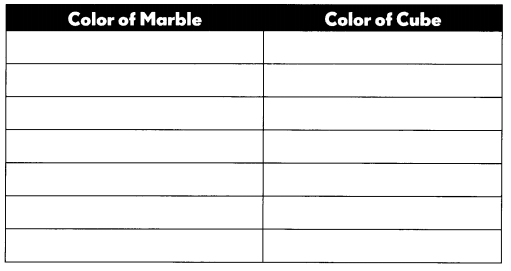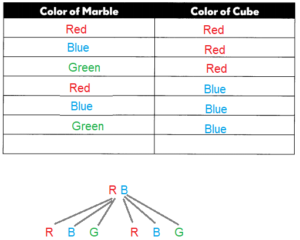Explanation:
A probability is a number that reflects the chance or likelihood that a particular event will occur.
A bag has 1 red, 1 blue, and 1 green marble.
Another bag has 1 red and 1 blue cube.
So, probability may vary from one to one.

Question 2.
There are ___________ combinations.
There are 6 combinations.
Explanation:
As we know probability is chance of occurrence,
so there are 6 possible combinations.

Complete.

In a soccer tournament, there are two groups. Each group has three teams. Teams A, B, and C are in Group 1. Teams X, Y, and Z are in Group 2. Each team in Group 1 plays against every team in Group 2.

Question 3.
Complete the table for the games played.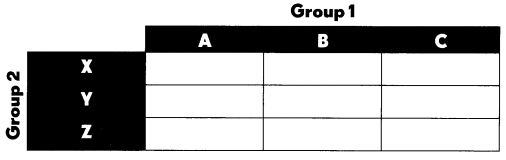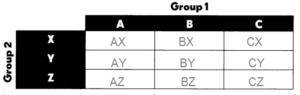Explanation:
In a soccer tournament, there are two groups.
Each group has three teams.
Teams A, B, and C are in Group 1.
Teams X, Y, and Z are in Group 2.
Each team in Group 1 plays against every team in Group 2.
As we know probability is chance of occurrence,
so there are 9 possible combinations.

Question 4.
The number of combinations of games for the six teams is ___.
9 combinations
Explanation:
As we know probability is chance of occurrence,
so there are 9 possible combinations of games for 6 teams.

Draw a tree diagram to find the number of combinations.

Question 5.
Ms. Li has 4 different books and 1 red pen, 1 blue pen, and 1 black pen. She is wrapping one book and one pen to give as a gift.
Draw a tree diagram to find the number of combinations she can choose.

There are ___ combinations.
12 combinations.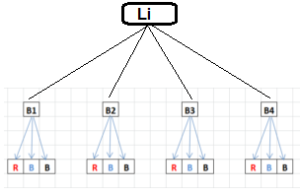Explanation:
Ms. Li has 4 different books.
She has 1 red pen, 1 blue pen, and 1 black pen.
She is wrapping one book and one pen to give as a gift.
As we know probability is chance of occurrence,
so there are 12 possible combinations.

Find the number of combinations.

Question 6.
Rina has 1 black, 1 red, and 1 yellow skirt. She has 1 white, 1 floral, and 1 striped shirt.

a. Draw a tree diagram to show the possible outfits Rina can wear.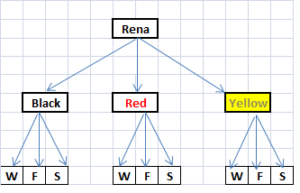Explanation:
A tree diagram is a tool in the fields of general mathematics, probability, and statistics.
It helps to calculate the number of possible outcomes of an event or problem,
and to cite those potential outcomes in an organized way.

b. Find the number of outfits by multiplication.
The number of outfits is ____.
The number of outfits is 9
Explanation:
Rina has 1 black, 1 red, and 1 yellow skirt.
She has 1 white, 1 floral, and 1 striped shirt.
All together she has 3 skirts and 3 shirts.
So, the number of outfits she can wear
3 x 3 = 9

Complete.

Question 7.
There are 4 colors on a spinner. There are 6 faces on a number cube, numbered 1 to 6. The spinner is spun and the number cube is tossed.
There are ___________ combinations of color and number.
24 combinations of color and number.
Explanation:
There are 24 combinations of color and number.
m x n is the possible combinations of color and number.
m = 4
n = 6
m x n = 4 x 6  = 24

Question 8.
A bookshelf has 10 mathematics books, 8 science books, and 12 history books.

a. There are ___ combinations of a mathematics book and a science book.
80 combinations of mathematics and science books.
Explanation:
There are 80 combinations of a mathematics book and a science book.
m x s is the possible combinations of a mathematics book and a science book.
m = 10
s  = 8
m x s = 10 x 8  = 80

b. There are ___________ combinations of a science book and a history book.